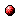# A modeling framework for model predictive scheduling using switching max-plus linear models

Reference:
T.J.J. van den Boom, G.D. Lopes, and B. De Schutter, "A modeling framework for model predictive scheduling using switching max-plus linear models," Proceedings of the 52nd IEEE Conference on Decision and Control, Florence, Italy, pp. 5456-5461, Dec. 2013.

Abstract:
In this paper we discuss a modeling framework for model predictive scheduling of a class of semi-cyclic discrete event systems that can be described by switching max-plus linear models. We study the structure of the system matrices and derive how routing, ordering, and synchronization can be manipulated by a set of control variables. In addition, we show that this leads to a system matrix that is linear in the control variables. We define the model predictive scheduling design problem to optimize the schedule, and we show that the problem can be recast as a mixed integer linear programming (MILP) problem.Corresponding technical report: pdf file (146 KB)
Note: More information on the pdf file format mentioned above can be found here.

Bibtex entry:

@inproceedings{vanLop:13-040,
author={T.J.J. van den Boom and G.D. Lopes and B. {D}e Schutter},
title={A modeling framework for model predictive scheduling using switching max-plus linear models},
booktitle={Proceedings of the 52nd IEEE Conference on Decision and Control},